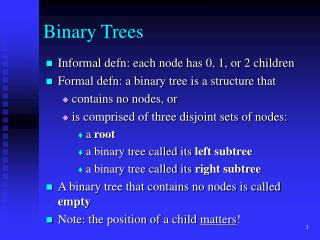DownloadDownload PresentationBinary Trees

# Binary Trees

Download Presentation## Binary Trees

- - - - - - - - - - - - - - - - - - - - - - - - - - - E N D - - - - - - - - - - - - - - - - - - - - - - - - - - -
##### Presentation Transcript

1. Binary Trees • Informal defn: each node has 0, 1, or 2 children • Formal defn: a binary tree is a structure that • contains no nodes, or • is comprised of three disjoint sets of nodes: • a root • a binary tree called its left subtree • a binary tree called its right subtree • A binary tree that contains no nodes is called empty • Note: the position of a child matters!

2. Binary Trees • Full binary tree : • All internal nodes have two children. • Complete binary tree : • All leaves have the same depth • All internal nodes have two children • A complete binary tree of height h has 2h-1 internal nodes and 2h leaves • Also: a binary tree with n nodes hasheight at least  lgn 

3. Tree applications • Expression evaluations (note how different traversals result in different notation) • Parsing (as part of the compilation process) • Storing and retrieving information by a key • Representing structured objects (e.g. the universe in an adventure game) • Useful when needing to make a decision on how to proceed (tic-tac-toe, chess)

4. Binary tree traversal • Traversing a tree = visiting its nodes • There are three ways to traverse a binary tree • preorder : visit root, visit left subtree, visit right subtree • inorder : visit left subtree, visit root, visit right subtree • postorder : visit left subtree, visit right subtree, visit root

5. Example: Inorder traversal template <class T> Tree<T>::Inorder(TreeNode<T> *subroot ) { if (subroot!=NULL) { Inorder(subroot  left); cout << subrootelement; Inorder(subroot right); } }

6. Binary trees • Use for storing and retrieving information • We want to insert, delete and search at least as fast and, if possible, faster than with a linked list • We want to take advantage of the lgn height • Idea: Store information in an ordered way (use a key) • Result: a Binary Search Tree (BST)

7. Binary Search Tree (BST) • A BST is a binary tree with the following property: • The key of the root is larger than any key in the left subtree and smaller than any key in the right subtree (the subtrees are also BSTs) • Note: This definition does not allow duplicate keys. • It’s now easy to search for an element

8. Binary Search Tree (BST) • How do we insert an element into a BST? • We have to make sure it is inserted at the correct position. • It is a combination of Search and Insert.

9. Binary Search Tree (BST) • How do we remove an element from a BST? • Removing a leaf is easy • Removing an internal node can be tricky. • The tree will need to be rearranged(how?)

10. Balanced trees • The good news: Why not sort a sequence by inserting the elements into a BST? • on average O(nlgn) comparisons • we get to keep the tree • The bad news: The insertion procedure can result in a tree of height n after inserting n elements. • We would prefer to get trees that are guaranteed to have logarithmic height in the worst case. • Such trees are called balanced.

11. AVL trees • AVL tree = a binary search tree with the following property: for every node the heights of the left and right subtrees differ at most by one. • That’s all very nice but how do we guarantee it? • We have to somehow modify the insert and delete functions. • If, after an insertion or deletion, the property is not satisfied, we “rotate” the tree to make it balanced.

12. AVL trees • When can an insertion of a child y at node x cause an imbalance? • when both x and y are left children • when both x and y are right children • when x is a right child and y is a left child • when y is a right child and x is a left child

13. AVL trees • There are two types of rotations: • single rotation • fixes imbalance of type 1/2 • double rotation • fixes imbalance of type 3/4 • Let’s draw some trees...

14. AVL trees • How/when do we decide whether to rotate? • Example: insertion • step 1: walk down the tree to insert the node in the correct position • step 2: walk up the tree checking the property at each node • we need a helper function to determine the heights of the subtrees of each node. • we need to be able to determine whether to perform a single or a double rotation

15. AVL trees • Just how balanced are AVL trees? • It can be shown that the worst case height of an AVL tree is at most 44% more than the minimum possible for BST (i.e. approximately 1.44 lgn) • What does this mean? • Searching/Inserting/Removing now take O(lgn) in the worst case.